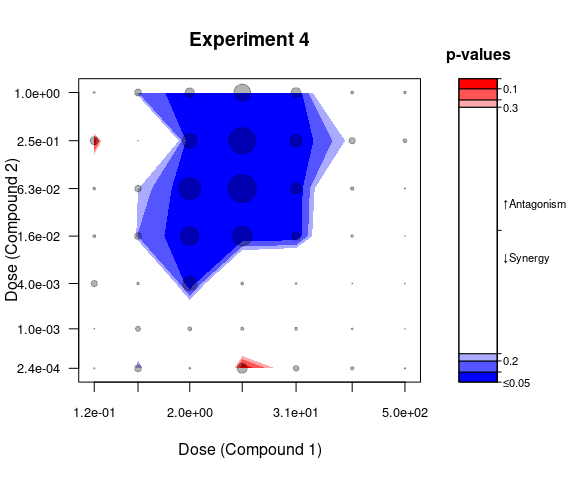# Synergy analysis

#### 2022-05-04

We first load the necessary packages and set some pre-defined values needed to replicate the analysis.

library(BIGL)
library(knitr)
library(rgl)
library(ggplot2)
set.seed(12345)

if (!requireNamespace("rmarkdown", quietly = TRUE) || !rmarkdown::pandoc_available("1.14")) {
warning(call. = FALSE, "These vignettes assume rmarkdown and pandoc
knitr::knit_exit()
}
nExp <- 4             # Dataset has 11 experiments, we consider only 4
cutoff <- 0.95        # Cutoff for p-values to use in plot.maxR() function

## Process and clean the data

The data for the analysis must come in a data-frame with required columns d1, d2 and effect for doses of two compounds and observed cell counts respectively. The effect column may represent also a type of normalized data and subsequent transformation functions should be adjusted.

We will use sample data included in the package - directAntivirals.

data("directAntivirals", package = "BIGL")
head(directAntivirals)
##   experiment   cpd1   cpd2 effect  d1  d2
## 1          1 cpd1_A cpd2_A 509.64 500 500
## 2          1 cpd1_A cpd2_A 589.67 500 500
## 3          1 cpd1_A cpd2_A 524.71 500 130
## 4          1 cpd1_A cpd2_A 575.45 500 130
## 5          1 cpd1_A cpd2_A 634.53 500  31
## 6          1 cpd1_A cpd2_A 702.35 500  31

This data consists of 11 experiments that can be processed separately. For initial illustration purposes we choose just one experiment and retain only the columns of interest. We define a simple function to do just that.

subsetData <- function(data, i) {
## Subset data to a single experiment and, optionally, select the necessary
## columns only
subset(data, experiment == i)[, c("effect", "d1", "d2")]
}

Now let us only pick Experiment 1 to illustrate the functionality of the package.

i <- 1
data <- subsetData(directAntivirals, i)

Dose-response data for Experiment 1 will be used for a large share of the analysis presented here, therefore this subset is stored in a dataframe called data. Later, we will run the analysis for some other experiments as well.

## Data transformation

If raw data is measured in cell counts, data transformation might be of interest to improve accuracy and interpretation of the model. Of course, this will depend on the model specification. For example, if a generalized Loewe model is assumed on the growth rate of the cell count, the appropriate conversion should be made from the observed cell counts. The formula used would be $y = N_0\exp\left(kt\right)$ where $$k$$ is a growth rate, $$t$$ is time (fixed) and $$y$$ is the observed cell count. If such a transformation is specified, it is referred to as the biological transformation.

In certain cases, variance-stabilizing transformations (Box-Cox) can also be useful. We refer to these transformations as power transformations. In many cases, a simple logarithmic transformation can be sufficient but, if desired, a helper function optim.boxcox is available to automate the selection of Box-Cox transformation parameters.

In addition to specifying biological and power transformations, users are also asked to specify their inverses. These are later used in the bootstrapping procedure and plotting methods.

As an example, we might define a transforms list that will be passed to the fitting functions. It contains both biological growth rate and power transformations along with their inverses.

## Define forward and reverse transform functions
transforms <- list(
"BiolT" = function(y, args) with(args, N0*exp(y*time.hours)),
"InvBiolT" = function(T, args) with(args, 1/time.hours*log(T/N0)),
"PowerT" = function(y, args) with(args, log(y)),
"InvPowerT" = function(T, args) with(args, exp(T)),
"compositeArgs" = list(N0 = 1,
time.hours = 72)
)

compositeArgs contains the initial cell counts (N0) and incubation time (time.hours). In certain cases, the getTransformations wrapper function can be employed to automatically obtain a prepared list with biological growth rate and power transformations based on results from optim.boxcox. Its output will also contain the inverses of these transforms.

transforms_auto <- getTransformations(data)
fitMarginals(data, transforms = transforms_auto)

## In the case of 1-parameter Box-Cox transformation, it is easy
## to retrieve the power parameter by evaluating the function at 0.
## If parameter is 0, then it is a log-transformation.
with(transforms_auto, -1 / PowerT(0, compositeArgs))

# Analysis

Once dose-response dataframe is correctly set up, we may proceed onto synergy analysis. We will use transforms as defined above with a logarithmic transformation. If not desired, transforms can be set to NULL and would be ignored.

Synergy analysis is quite modular and is divided into 3 parts:

1. Determine marginal curves for each of the compounds. These curves are computed based on monotherapy data, i.e. those observations where one of the compounds is dosed at 0.
2. Compute expected effects for a chosen null model given the previously determined marginal curves at various dose combinations.
3. Compare the expected response with the observed effect using statistical testing procedures.

## Fitting marginal (on-axis) data

The first step of the fitting procedure will consist in treating marginal data only, i.e. those observations within the experiment where one of the compounds is dosed at zero. For each compound the corresponding marginal doses are modelled using a 4-parameter logistic model.

The marginal models will be estimated together using non-linear least squares estimation procedure. Estimation of both marginal models needs to be simultaneous since it is assumed they share a common baseline that also needs to be estimated. The fitMarginals function and other marginal estimation routines will automatically extract marginal data from the dose-response data frame.

Before proceeding onto the estimation, we get a rough guess of the parameters to use as starting values in optimization and then we fit the model. marginalFit, returned by the fitMarginals routine, is an object of class MarginalFit which is essentially a list containing the main information about the marginal models, in particular the estimated coefficients.

The optional names argument allows to specify the names of the compounds to be shown on the plots and in the summary. If not defined, the defaults (“Compound 1” and “Compound 2”) are used.

## Fitting marginal models
marginalFit <- fitMarginals(data, transforms = transforms, method = "nls",
names = c("Drug A", "Drug B"))
summary(marginalFit)
## Formula: effect ~ fn(h1, h2, b, m1, m2, e1, e2, d1, d2)
## Transformations: Yes
##
##                  Drug A Drug B
## Slope             2.132  1.201
## Maximal response  0.088  0.091
## log10(EC50)       1.008  0.977
##
## Common baseline at: 0.132

marginalFit object retains the data that was supplied and the transformation functions used in the fitting procedure. It also has a plot method which allows for a quick visualization of the fitting results.

## Plotting marginal models
plot(marginalFit) + ggtitle(paste("Direct-acting antivirals - Experiment" , i))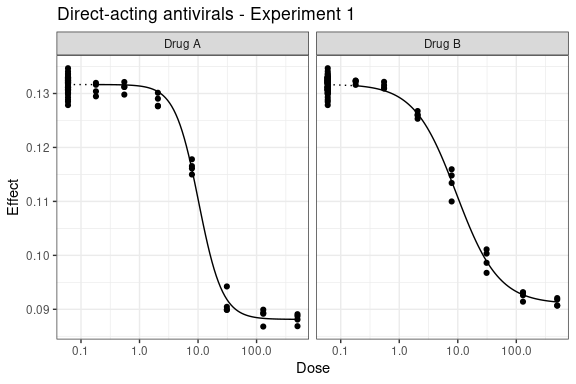Note as well that the fitMarginals function allows specifying linear constraints on parameters. This provides an easy way for the user to impose asymptote equality, specific baseline value and other linear constraints that might be useful. See help(constructFormula) for more details.

## Parameter ordering: h1, h2, b, m1, m2, e1, e2
## Constraint 1: m1 = m2. Constraint 2: b = 10.
constraints <- list("matrix" = rbind(c(0, 0, 0, -1, 1, 0, 0),
c(0, 0, 1, 0, 0, 0, 0)),
"vector" = c(0, 10))

## Parameter estimates will now satisfy equality:
##   constraints$matrix %*% pars == constraints$vector
fitMarginals(data, transforms = transforms,
constraints = constraints)

The fitMarginals function allows an alternative user-friendly way to specify one or more fixed-value constraints using a named vector passed to the function via fixed argument.

## Set baseline at 1 and maximal responses at 0.
fitMarginals(data, transforms = transforms,
fixed = c("m1" = 0, "m2" = 0, "b" = 1))

By default, no constraints are set, thus asymptotes are not shared and so a generalized Loewe model will be estimated.

### Optimization algorithms

We advise the user to employ the method = "nlslm" argument which is set as the default in monotherapy curve estimation. It is based on minpack.lm::nlsLM function with an underlying Levenberg-Marquardt algorithm for non-linear least squares estimation. This algorithm is known to be more robust than method = "nls" and its Gauss-Newton algorithm. In cases with nice sigmoid-shaped data, both methods should however lead to similar results.

method = "optim" is a simple sum-of-squared-residuals minimization driven by a default Nelder-Mead algorithm from optim minimizer. It is typically slower than non-linear least squares based estimation and can lead to a significant increase in computational time for larger datasets and bootstrapped statistics. In nice cases, Nelder-Mead algorithm and non-linear least squares can lead to rather similar estimates but this is not always the case as these algorithms are based on different techniques.

In general, we advise that in automated batch processing whenever method = "nlslm" does not converge fast enough and/or emits a warning, user should implement a fallback to method = "optim" and re-do the estimation. If none of these suggestions work, it might be useful to fiddle around and slightly perturb starting values for the algorithms as well. By default, these are obtained from the initialMarginal function.

nlslmFit <- tryCatch({
fitMarginals(data, transforms = transforms,
method = "nlslm")
}, warning = function(w) w, error = function(e) e)

if (inherits(nlslmFit, c("warning", "error")))
optimFit <- tryCatch({
fitMarginals(data, transforms = transforms,
method = "optim")
})

Note as well that additional arguments to fitMarginals passed via ... ellipsis argument will be passed on to the respective solver function, i.e. minpack.lm::nlsLM, nls or optim.

### Custom marginal fit

While BIGL package provides several routines to fit 4-parameter log-logistic dose-response models, some users may prefer to use their own optimizers to estimate the relevant parameters. It is rather easy to integrate this into the workflow by constructing a custom MarginalFit object. It is in practice a simple list with

• coef: named vector with coefficient estimates
• sigma: standard deviation of residuals
• df: degrees of freedom from monotherapy curve estimates
• model: model of the marginal estimation which allows imposing linear constraints on parameters. If no constraints are necessary, it can be left out or assigned the output of constructFormula function with no inputs.
• shared_asymptote: whether estimation is constrained to share the asymptote. During the estimation, this is deduced from model object.
• method: method used in dose-response curve estimation which will be re-used in bootstrapping
• transforms: power and biological transformation functions (and their inverses) used in monotherapy curve estimation. This should be a list in a format described above. If transforms is unspecified or NULL, no transformations will be used in statistical bootstrapping unless the user asks for it explicitly via one of the arguments to fitSurface.

Other elements in the MarginalFit are currently unused for evaluating synergy and can be disregarded. These elements, however, might be necessary to ensure proper working of available methods for the MarginalFit object.

As an example, the following code generates a custom MarginalFit object that can be passed further to estimate a response surface under the null hypothesis.

marginalFit <- list("coef" = c("h1" = 1, "h2" = 2, "b" = 0,
"m1" = 1.2, "m2" = 1, "e1" = 0.5, "e2" = 0.5),
"sigma" = 0.1,
"df" = 123,
"model" = constructFormula(),
"shared_asymptote" = FALSE,
"method" = "nlslm",
"transforms" = transforms)
class(marginalFit) <- append(class(marginalFit), "MarginalFit")

Note that during bootstrapping this would use minpack.lm::nlsLM function to re-estimate parameters from data following the null. A custom optimizer for bootstrapping is currently not implemented.

## Compute expected response for off-axis data

Five types of null models are available for calculating expected response surfaces.

• Generalized Loewe model is used if maximal responses are not constrained to be equal, i.e. shared_asymptote = FALSE, in the marginal fitting procedure and null_model = "loewe" in response calculation.
• Classical Loewe model is used if constraints are such that shared_asymptote = TRUE in the marginal fitting procedure and null_model = "loewe" in response calculation.
• Highest Single Agent is used if null_model = "hsa" irrespective of the value of shared_asymptote.
• Bliss independence model is used when null_model = "bliss". In the situations when maximal responses are constrained to be equal, the classical Bliss independence approach is used, when they are not equal, the Bliss independence calculation is performed on responses rescaled to the maximum range (i.e. absolute difference between baseline and maximal response).
• Alternative Loewe Generalization is used when null_model = "loewe2". If the asymptotes are constrained to be equal, this reduces to the classical Loewe. Note that if shared_asymptote = TRUE constraints are used, this also reduces to classical Loewe model.

### (Generalized) Loewe model

If transformation functions were estimated using fitMarginals, these will be automatically recycled from the marginalFit object when doing calculations for the response surface fit. Alternatively, transformation functions can be passed by a separate argument. Since the marginalFit object was estimated without the shared asymptote constraint, the following will compute the response surface based on the generalized Loewe model.

rs <- fitSurface(data, marginalFit,
null_model = "loewe",
B.CP = 50, statistic = "none", parallel = FALSE)
summary(rs)
Null model: Generalized Loewe Additivity
Variance assumption used: "equal"
Mean occupancy rate: 0.6732745

Formula: effect ~ fn(h1, h2, b, m1, m2, e1, e2, d1, d2)
Transformations: Yes

Drug A Drug B
Slope             2.132  1.201
Maximal response  0.088  0.091
log10(EC50)       1.008  0.977

Common baseline at: 0.132

No test statistics were computed.
CONFIDENCE INTERVALS
Overall effect
Estimated mean departure from null response surface with 95% confidence interval:
-0.0668 [-0.113, -0.0203]
Evidence for effects in data: Syn

Significant pointwise effects
estimate   lower   upper call
2_7.8    -0.2162 -0.4239 -0.0085  Syn
7.8_31   -0.2770 -0.4768 -0.0773  Syn
7.8_7.8  -0.5354 -0.7488 -0.3220  Syn

Pointwise 95% confidence intervals summary:
Syn Ant Total
3   0    49

The occupancy matrix used in the expected response calculation for the Loewe models can be accessed with rs$occupancy. For off-axis data and a fixed dose combination, the Z-score for that dose combination is defined to be the standardized difference between the observed effect and the effect predicted by a generalized Loewe model. If the observed effect differs significantly from the prediction, it might be due to the presence of synergy or antagonism. If multiple observations refer to the same combination of doses, then a mean is taken over these multiple standardized differences. The following plot illustrates the isobologram of the chosen null model. Coloring and contour lines within the plot should help the user distinguish areas and dose combinations that generate similar response according to the null model. Note that the isobologram is plotted by default on a logarithmically scaled grid of doses. isobologram(rs)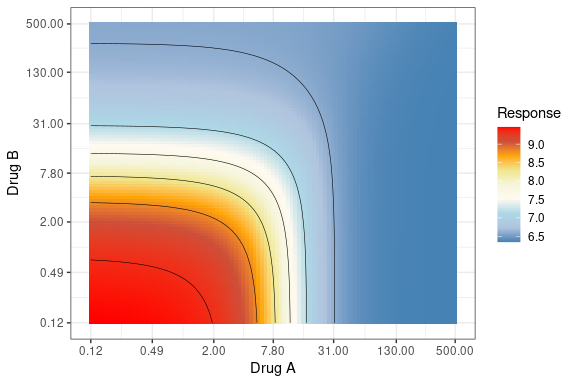The plot below illustrates the above considerations in a 3-dimensional setting. In this plot, points refer to the observed effects whereas the surface is the model-predicted response. The surface is colored according to the median Z-scores where blue coloring indicates possible synergistic effects (red coloring would indicate possible antagonism). plot(rs, legend = FALSE, main = "") view3d(0, -75) rglwidget() ### Highest Single Agent For the Highest Single Agent null model to work properly, it is expected that both marginal curves are either decreasing or increasing. Equivalent summary and plot methods are also available for this type of null model. rsh <- fitSurface(data, marginalFit, null_model = "hsa", B.CP = 50, statistic = "both", parallel = FALSE) summary(rsh) Null model: Highest Single Agent with differing maximal response Variance assumption used: "equal" Formula: effect ~ fn(h1, h2, b, m1, m2, e1, e2, d1, d2) Transformations: Yes Drug A Drug B Slope 2.132 1.201 Maximal response 0.088 0.091 log10(EC50) 1.008 0.977 Common baseline at: 0.132 Exact meanR test (H0 = no synergy/antagonism): F(49,117) = 17.2006 (p-value < 2e-16) Evidence for effects in data: Syn Points with significant deviations from the null: d1 d2 absR p-value call 2_0.12 2.0 0.12 4.764083 0.00015 Syn 2_0.49 2.0 0.49 4.606532 0.00039 Syn 2_2 2.0 2.00 4.320753 0.00148 Syn 2_7.8 2.0 7.80 4.595625 0.00041 Syn 7.8_2 7.8 2.00 8.539806 <2e-16 Syn 7.8_31 7.8 31.00 8.173404 <2e-16 Syn 7.8_7.8 7.8 7.80 22.495627 <2e-16 Syn Overall maxR summary: Call Syn Ant Total Syn 7 0 49 CONFIDENCE INTERVALS Overall effect Estimated mean departure from null response surface with 95% confidence interval: -0.1219 [-0.1626, -0.0808] Evidence for effects in data: Syn Significant pointwise effects estimate lower upper call 0.49_7.8 -0.2232 -0.4125 -0.0339 Syn 2_0.12 -0.2193 -0.3926 -0.0459 Syn 2_0.49 -0.2239 -0.3928 -0.0549 Syn 2_2 -0.3246 -0.5196 -0.1295 Syn 2_31 -0.2554 -0.4484 -0.0624 Syn 2_7.8 -0.4256 -0.6149 -0.2364 Syn 31_500 -0.2312 -0.4237 -0.0388 Syn 7.8_130 -0.1852 -0.3671 -0.0034 Syn 7.8_2 -0.5581 -0.7603 -0.3559 Syn 7.8_31 -0.5155 -0.7085 -0.3225 Syn 7.8_7.8 -1.2862 -1.4755 -1.0970 Syn Pointwise 95% confidence intervals summary: Syn Ant Total 11 0 49 ### Bliss Independence Also for the Bliss independence null model to work properly, it is expected that both marginal curves are either decreasing or increasing. Equivalent summary and plot methods are also available for this type of null model. rsb <- fitSurface(data, marginalFit, null_model = "bliss", B.CP = 50, statistic = "both", parallel = FALSE) summary(rsb) Null model: Bliss independence with differing maximal response Variance assumption used: "equal" Formula: effect ~ fn(h1, h2, b, m1, m2, e1, e2, d1, d2) Transformations: Yes Drug A Drug B Slope 2.132 1.201 Maximal response 0.088 0.091 log10(EC50) 1.008 0.977 Common baseline at: 0.132 Exact meanR test (H0 = no synergy/antagonism): F(49,117) = 4.4493 (p-value = 0) Evidence for effects in data: Syn Points with significant deviations from the null: d1 d2 absR p-value call 2_7.8 2.0 7.8 4.496210 0.00089 Syn 7.8_7.8 7.8 7.8 9.325958 <2e-16 Syn Overall maxR summary: Call Syn Ant Total Syn 2 0 49 CONFIDENCE INTERVALS Overall effect Estimated mean departure from null response surface with 95% confidence interval: -0.0688 [-0.1013, -0.0232] Evidence for effects in data: Syn Significant pointwise effects estimate lower upper call 0.49_7.8 -0.2203 -0.4272 -0.0135 Syn 2_0.12 -0.2044 -0.3861 -0.0228 Syn 2_2 -0.2417 -0.4505 -0.0330 Syn 2_31 -0.2320 -0.4476 -0.0163 Syn 2_7.8 -0.3700 -0.5824 -0.1576 Syn 7.8_2 -0.3088 -0.5369 -0.0806 Syn 7.8_31 -0.2347 -0.4306 -0.0388 Syn 7.8_7.8 -0.6198 -0.8267 -0.4130 Syn Pointwise 95% confidence intervals summary: Syn Ant Total 8 0 49 ### Alternative Loewe Generalization Also for the Alternative Loewe Generalization null model to work properly, it is expected that both marginal curves are either decreasing or increasing. Equivalent summary and plot methods are also available for this type of null model. rsl2 <- fitSurface(data, marginalFit, null_model = "loewe2", B.CP = 50, statistic = "both", parallel = FALSE) summary(rsl2) Null model: Alternative generalization of Loewe Additivity Variance assumption used: "equal" Formula: effect ~ fn(h1, h2, b, m1, m2, e1, e2, d1, d2) Transformations: Yes Drug A Drug B Slope 2.132 1.201 Maximal response 0.088 0.091 log10(EC50) 1.008 0.977 Common baseline at: 0.132 Exact meanR test (H0 = no synergy/antagonism): F(49,117) = 4.6653 (p-value = 0) Evidence for effects in data: Syn Points with significant deviations from the null: d1 d2 absR p-value call 2_0.12 2.0 0.12 3.845699 0.01033 Syn 7.8_31 7.8 31.00 4.458567 0.00091 Syn 7.8_7.8 7.8 7.80 9.123321 <2e-16 Syn Overall maxR summary: Call Syn Ant Total Syn 3 0 49 CONFIDENCE INTERVALS Overall effect Estimated mean departure from null response surface with 95% confidence interval: -0.0706 [-0.1061, -0.0387] Evidence for effects in data: Syn Significant pointwise effects estimate lower upper call 7.8_31 -0.2998 -0.5403 -0.0594 Syn 7.8_7.8 -0.5565 -0.8128 -0.3003 Syn Pointwise 95% confidence intervals summary: Syn Ant Total 2 0 49 ## Statistical testing Presence of synergistic or antagonistic effects can be formalized by means of statistical tests. Two types of tests are considered here and are discussed in more details in the methodology vignette as well as the accompanying paper. • meanR test evaluates how the predicted response surface based on a specified null model differs from the observed one. If the null hypothesis is rejected, this test suggests that at least some dose combinations may exhibit synergistic or antagonistic behaviour. The meanR test is not designed to pinpoint which combinations produce these effects nor what type of deviating effect is present. • maxR test allows to evaluate presence of synergistic/antagonistic effects for each dose combination and as such provides a point-by-point classification. Both of the above test statistics have a well specified null distribution under a set of assumptions, namely normality of Z-scores. If this assumption is not satisfied, distribution of these statistics can be estimated using bootstrap. Normal approximation is significantly faster whereas bootstrapped distribution of critical values is likely to be more accurate in many practical cases. ### meanR Here we will use the previously computed CP covariance matrix to speed up the process. • normal errors meanR_N <- fitSurface(data, marginalFit, statistic = "meanR", CP = rs$CP, B.B = NULL,
parallel = FALSE)
• non-normal errors

The previous piece of code assumes normal errors. If we drop this assumption, we can use bootstrap methods to resample from the observed errors. Other parameters for bootstrapping, such as additional distribution for errors, wild bootstrapping to account for heteroskedasticity, are also available. See help(fitSurface).

meanR_B <- fitSurface(data, marginalFit,
statistic = "meanR", CP = rs$CP, B.B = 20, parallel = FALSE) Both tests use the same calculated F-statistic but compare it to different null distributions. In this particular case, both tests lead to identical results. F-statistic p-value Normal errors 4.353656 0 Bootstrapped errors 4.353656 0 ### maxR The meanR statistic can be complemented by the maxR statistic for each of available dose combinations. We will do this once again by assuming both normal and non-normal errors similar to the computation of the meanR statistic. maxR_N <- fitSurface(data, marginalFit, statistic = "maxR", CP = rs$CP, B.B = NULL,
parallel = FALSE)
maxR_B <- fitSurface(data, marginalFit,
statistic = "maxR", CP = rs$CP, B.B = 20, parallel = FALSE) maxR_both <- rbind(summary(maxR_N$maxR)$totals, summary(maxR_B$maxR)$totals) Here is the summary of maxR statistics. It lists the total number of dose combinations listed as synergistic or antagonistic for Experiment 1 given the above calculations. Call Syn Ant Total Normal errors Syn 3 0 49 Bootstrapped errors Syn 2 0 49 By using the outsidePoints function, we can obtain a quick summary indicating which dose combinations in Experiment 1 appear to deviate significantly from the null model according to the maxR statistic. outPts <- outsidePoints(maxR_B$maxR$Ymean) kable(outPts, caption = paste0("Non-additive points for Experiment ", i)) Non-additive points for Experiment 1 d1 d2 absR p-value call 7.8_31 7.8 31.0 4.176872 0.06 Syn 7.8_7.8 7.8 7.8 8.905776 0.00 Syn Synergistic effects of drug combinations can be depicted in a bi-dimensional contour plot where the x-axis and y-axis represent doses of Compound 1 and Compound 2 respectively and each point is colored based on the p-value and sign of the respective maxR statistic. contour(maxR_B, ## colorPalette = c("blue", "black", "black", "red"), main = paste0(" Experiment ", i, " contour plot for maxR"), scientific = TRUE, digits = 3, cutoff = cutoff)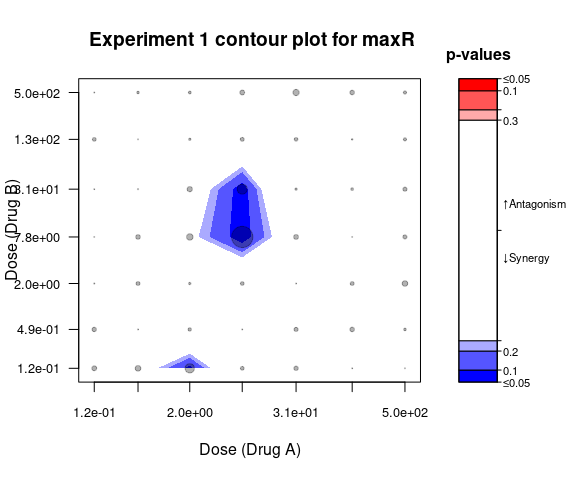Previously, we had colored the 3-dimensional predicted response surface plot based on its Z-score, i.e. deviation of the predicted versus the observed effect. We can also easily color it based on the computed maxR statistic to account for additional statistical variation. plot(maxR_B, color = "maxR", legend = FALSE, main = "") view3d(0, -75) rglwidget() ### Effect sizes and confidence interval The BIGL package also yields effect sizes and corresponding confidence intervals with respect to any response surface. The overall effect size and confidence interval is output in the summary of the ResponseSurface, but can also be called directly: summary(maxR_B$confInt)
## Overall effect
## Estimated mean departure from null response surface with 95% confidence interval:
## -0.0668 [-0.099, -0.0242]
## Evidence for effects in data: Syn
##
## Significant pointwise effects
##         estimate   lower   upper call
## 7.8_31   -0.2770 -0.4858 -0.0683  Syn
## 7.8_7.8  -0.5354 -0.7584 -0.3124  Syn
##
## Pointwise 95% confidence intervals summary:
##  Syn Ant Total
##    2   0    49

In addition, a contour plot can be made with pointwise confidence intervals. Contour plot colouring can be defined according to the effect sizes or according to maxR results.

plotConfInt(maxR_B, color = "effect-size")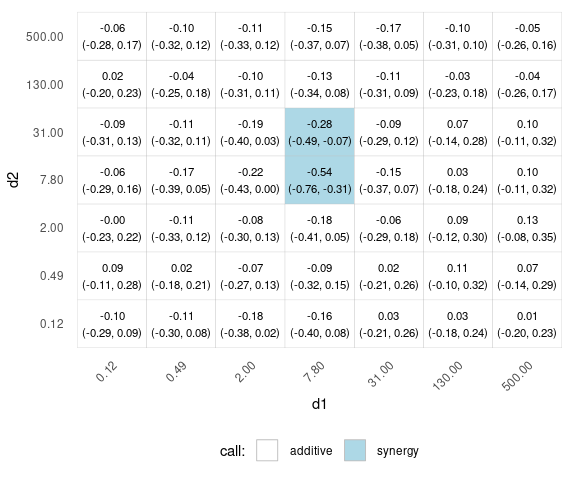# Analysis in case of variance heterogeneity

Starting from the package version 1.2.0 the variance can be estimated separately for on-axis (monotherapy) and off-axis points using method argument to fitSurface. The possible values for method are:

• "equal", equal variances assumed (as above, default),
• "unequal", variance is estimated separately for on-axis and off-axis points,
• "model", the variance is modelled as a function of the mean.

Please see the methodology vignette for details. Below we show an example analysis in such case. Note that transformations are not possible if variances are not assumed equal.

marginalFit <- fitMarginals(data, transforms = NULL)
summary(marginalFit)
## Formula: effect ~ fn(h1, h2, b, m1, m2, e1, e2, d1, d2)
## Transformations: No
##
##                  Compound 1 Compound 2
## Slope                 1.579      1.459
## Maximal response    425.607    789.029
## log10(EC50)           0.663      0.500
##
## Common baseline at: 13200.55
resU <- fitSurface(data, marginalFit, method = "unequal",
statistic = "both", B.CP = 20, B.B = 20, parallel = FALSE)
summary(resU)
## Null model: Generalized Loewe Additivity
## Variance assumption used: "unequal"
## Mean occupancy rate: 0.7663414
##
## Formula: effect ~ fn(h1, h2, b, m1, m2, e1, e2, d1, d2)
## Transformations: No
##
##                  Compound 1 Compound 2
## Slope                 1.579      1.459
## Maximal response    425.607    789.029
## log10(EC50)           0.663      0.500
##
## Common baseline at: 13200.55
##
## Bootstrapped meanR test (H0 = no synergy/antagonism):
##  F(49,117) = 3.9581 (p-value < 2e-16)
##
## Evidence for effects in data: Syn
## Points with significant deviations from the null:
##             d1   d2     absR p-value call
## 0.12_0.12 0.12 0.12 7.129077  <2e-16  Syn
## 0.49_0.12 0.49 0.12 6.520855  <2e-16  Syn
##
## Overall maxR summary:
##  Call Syn Ant Total
##   Syn   2   0    49
##
## CONFIDENCE INTERVALS
## Overall effect
## Estimated mean departure from null response surface with 95% confidence interval:
## -179.9317 [-777.8101, 27.9046]
## Evidence for effects in data: None
##
## Significant pointwise effects
##            estimate     lower     upper call
## 0.12_0.12 -1325.992 -2323.329 -328.6560  Syn
## 0.49_0.12 -1195.634 -2215.949 -175.3202  Syn
##
## Pointwise 95% confidence intervals summary:
##  Syn Ant Total
##    2   0    49

For the variance model, an exploratory plotting function is available to explore the relationship between the mean and the variance.

plotMeanVarFit(data)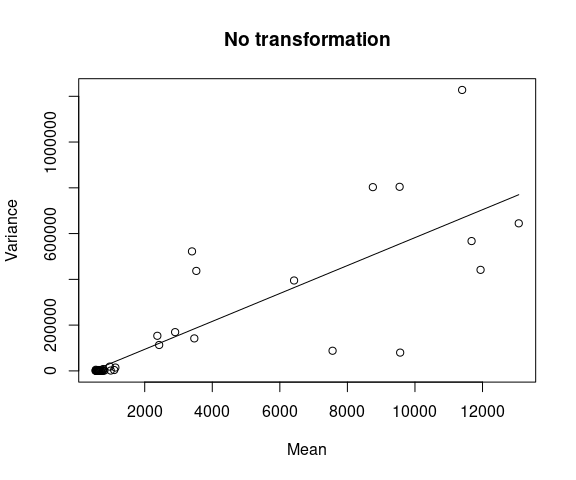plotMeanVarFit(data, log = "xy") #Clearer on the log-scale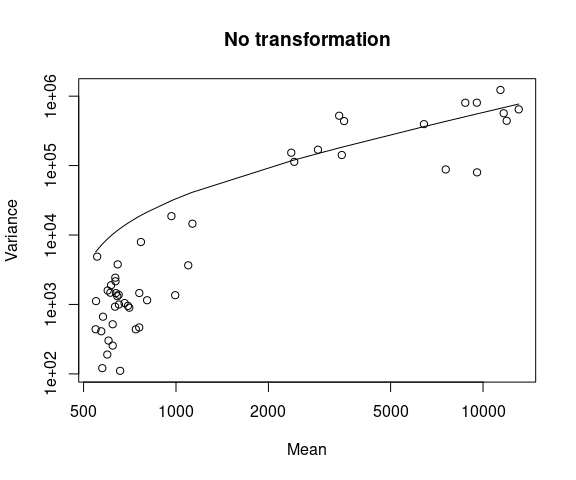plotMeanVarFit(data, trans = "log") #Thresholded at maximum observed variance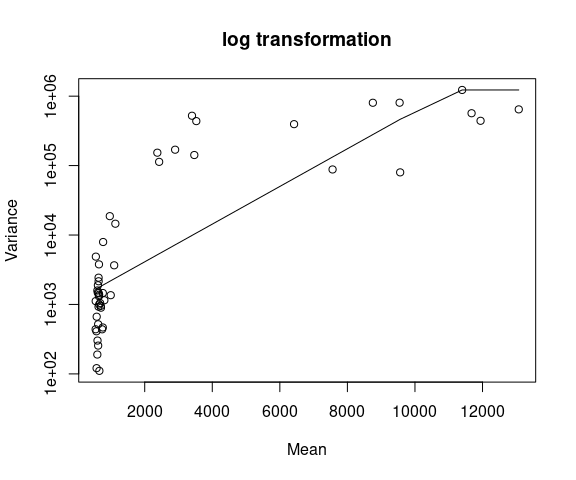The linear fit seems fine in this case.

resM <- fitSurface(data, marginalFit, method = "model",
statistic = "both", B.CP = 20, B.B = 20, parallel = FALSE)

If the log transformation yielded a better fit, then this could be achieved by using the following option.

resL <- fitSurface(data, marginalFit, method = "model", trans = "log",
statistic = "both", B.CP = 20, B.B = 20, parallel = FALSE)

Negative variances were modelled, but variance model has the smallest observed variances as minimum so we can proceed.

summary(resM) 
## Null model: Generalized Loewe Additivity
## Variance assumption used: "model"
## Mean occupancy rate: 0.7663414
##
## Formula: effect ~ fn(h1, h2, b, m1, m2, e1, e2, d1, d2)
## Transformations: No
##
##                  Compound 1 Compound 2
## Slope                 1.579      1.459
## Maximal response    425.607    789.029
## log10(EC50)           0.663      0.500
##
## Common baseline at: 13200.55
##
## Bootstrapped meanR test (H0 = no synergy/antagonism):
##  F(49,117) = 3.7381 (p-value < 2e-16)
##
## Evidence for effects in data: Syn
## Points with significant deviations from the null:
##          d1  d2     absR p-value call
## 7.8_7.8 7.8 7.8 7.951282  <2e-16  Syn
##
## Overall maxR summary:
##  Call Syn Ant Total
##   Syn   1   0    49
##
## CONFIDENCE INTERVALS
## Overall effect
## Estimated mean departure from null response surface with 95% confidence interval:
## -179.9317 [-509.0856, 14.7183]
## Evidence for effects in data: None
##
## Significant pointwise effects
##  estimate lower    upper    call
## <0 rows> (or 0-length row.names)
##
## Pointwise 95% confidence intervals summary:
##  Syn Ant Total
##    0   0    49

# Analysis of multiple experiments

In order to proceed with multiple experiments, we repeat the same procedure as previously. We collect all the necessary objects for which estimations do not have to be repeated to generate meanR and maxR statistics in a simple list.

marginalFits <- list()
datasets <- list()
respSurfaces <- list()
maxR.summary <- list()
for (i in seq_len(nExp)) {
## Select experiment
data <- subsetData(directAntivirals, i)
## Fit joint marginal model
marginalFit <- fitMarginals(data, transforms = transforms,
method = "nlslm")
## Predict response surface based on generalized Loewe model
respSurface <- fitSurface(data, marginalFit,
statistic = "maxR", B.CP = 20,
parallel = FALSE)

datasets[[i]] <- data
marginalFits[[i]] <- marginalFit
respSurfaces[[i]] <- respSurface
maxR.summary[[i]] <- summary(respSurface$maxR)$totals
}

We use the maxR procedure with a chosen p-value cutoff of 0.95. If maxR statistic falls outside the 95th percentile of its distribution (either bootstrapped or not), the respective off-axis dose combination is said to deviate significantly from the generalized Loewe model and the algorithm determines whether it deviates in a synergistic or antagonistic way.

Below is the summary of overall calls and number of deviating points for each experiment.

Call Syn Ant Total
Experiment 1 Syn 3 0 49
Experiment 2 Syn 5 0 49
Experiment 3 Syn 5 0 49
Experiment 4 Syn 13 2 49

Previous summarizing and visual analysis can be repeated on each of the newly defined experiments. For example, Experiment 4 indicates a total of 15 combinations that were called synergistic according to the maxR test.

d1 d2 absR p-value call
0.12_0.25 0.12 0.25000 3.369638 0.04933 Ant
2_0.004 2.00 0.00400 5.930847 0.00000 Syn
2_0.016 2.00 0.01600 7.503016 0.00000 Syn
2_0.063 2.00 0.06300 9.122209 0.00000 Syn
2_0.25 2.00 0.25000 6.078746 0.00000 Syn
2_1 2.00 1.00000 3.849097 0.00976 Syn
31_0.016 31.00 0.01600 3.480577 0.03389 Syn
31_0.063 31.00 0.06300 4.481778 0.00093 Syn
31_0.25 31.00 0.25000 5.174262 0.00003 Syn
31_1 31.00 1.00000 4.017347 0.00539 Syn
7.8_0.00024 7.80 0.00024 4.147400 0.00336 Ant
7.8_0.016 7.80 0.01600 8.180572 0.00000 Syn
7.8_0.063 7.80 0.06300 11.711154 0.00000 Syn
7.8_0.25 7.80 0.25000 10.980381 0.00000 Syn
7.8_1 7.80 1.00000 7.025138 0.00000 Syn

Consequently, above table for Experiment 4` can be illustrated in a contour plot.